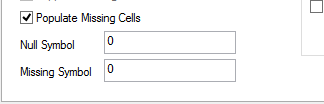# New to Qlik Sense

Discussion board where members can get started with Qlik Sense.

Announcements
Save \$300 - Register for QlikWorld (formerly Qonnections) by January 31st: Learn More
Highlighted
Contributor II

## replacing hypen with zero in table

Hi,

i have an expression where i am formatting column values in table. Now i need to replace hypen with 0, if value is null

Num(if(Metrics='Invitations',if(Sign(\$(=Count({\$<batch={'23','34'}>}invitation_id))=-1,0,\$(=Count({\$<batch={'23','34'}>}invitation_id)),

if(Metrics='Delivered',Alt(\$=(Sum(delivered)),0),

if(Metrics='Completions Rate',Sum(completions)/

Sum(delivered),))), if(Metrics='Completions Rate','#,##0.0%', '#,##0'))

How could i do this?

Tags (3)
1 Solution

Accepted Solutions
Contributor II

## Re: replacing hypen with zero in table

Hi guys,

first of all, i have to say sorry, because my expressions in query were not exactly same as i had posted and working expression.

i figured it out, why i was having this issue, the reason is

1.) i was using Dollar-sign Expansion with expression.

2.)If i need to use alt() or RangeMax() or sign() , i should not use Dollar-sign Expansion with expression, inside these(alt() or RangeMax() or sign()) functions.This may lead to undefined result, which was causing me to give hypen.

3.)If in single row, your expresion contains alt() or sign() function with dollar expansion, then all rows in that particular column can result as not valid, which will be shown in hypen symbol

My expression was

if(Sign(\$(=Count({\$<batch={'23','34'}>}invitation_id))=-1,0,\$(=Count({\$<batch={'23','34'}>}invitation_id))

Alt(\$=(Sum(delivered)),0)

In my above expression i should remove ' \$(= '  Dollar expansion symbol, then you get the result.

Thankyou all of you, to answering or giving hint for  my query.

16 RepliesMVP

## Re: replacing hypen with zero in table

Hi,

In a Chart properties set this settings Populate Missing CellsRegards

Anand

Contributor II

## Re: replacing hypen with zero in table

Hi,

its not a chart, it is table i need to do it in expression by putting condition.

Contributor III

## Re: replacing hypen with zero in table

If Sum(delivered) = 0, then Completions Rate= '-' (Any number / 0 will be shown as '-')

I think we need to address this as well.

Regards

HarishPartner

## Re: replacing hypen with zero in table

Try

Alt(Num(if(Metrics='Invitations',Count(invitation_id),

if(Metrics='Delivered',Sum(delivered),

if(Metrics='Completions Rate',Sum(completions)/

Sum(delivered),))), if(Metrics='Completions Rate','#,##0.0%', '#,##0')),0)

New Contributor III

## Re: replacing hypen with zero in table

A solution could be to use: RangeMax of RangeMin

RangeMax(0,

Num(if(Metrics='Invitations',Count(invitation_id),

if(Metrics='Delivered',Sum(delivered),

if(Metrics='Completions Rate',Sum(completions)/

Sum(delivered),))), if(Metrics='Completions Rate','#,##0.0%', '#,##0'))

Contributor II

## Re: replacing hypen with zero in table

Hi,

using of alt will work fine in text box, but not working in table.Why is it happening soMVP

## Re: replacing hypen with zero in table

So, Where is this expression you are using

Num(if(Metrics='Invitations',Count(invitation_id),

if(Metrics='Delivered',Sum(delivered),

if(Metrics='Completions Rate',Sum(completions)/

Sum(delivered),))), if(Metrics='Completions Rate','#,##0.0%', '#,##0'))

Or

In the Load script you required to do the manipulation for the NULL handling technique

https://community.qlik.com/docs/DOC-3155

Regards

Anand

Valued Contributor II

## Re: replacing hypen with zero in table

Hi Supriya

Better you bring this expression from back end

it could give you solution

Thanks,

ram

Contributor III

## Re: replacing hypen with zero in table

Supriya u can use IFNull(..(..(